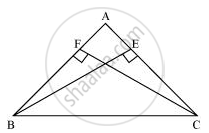# BE and CF are two equal altitudes of a triangle ABC. Using RHS congruence rule, prove that the triangle ABC is isosceles. - Mathematics

BE and CF are two equal altitudes of a triangle ABC. Using RHS congruence rule, prove that the triangle ABC is isosceles.

#### SolutionIn ΔBEC and ΔCFB,

∠BEC = ∠CFB (Each 90°)

BC = CB (Common)

BE = CF (Given)

∴ ΔBEC ≅ ΔCFB (By RHS congruency)

⇒ ∠BCE = ∠CBF (By CPCT)

∴ AB = AC (Sides opposite to equal angles of a triangle are equal)

Hence, ΔABC is isosceles.

Concept: Some More Criteria for Congruence of Triangles
Is there an error in this question or solution?
Chapter 7: Triangles - Exercise 7.3 [Page 128]

#### APPEARS IN

NCERT Class 9 Maths
Chapter 7 Triangles
Exercise 7.3 | Q 4 | Page 128

Share